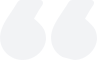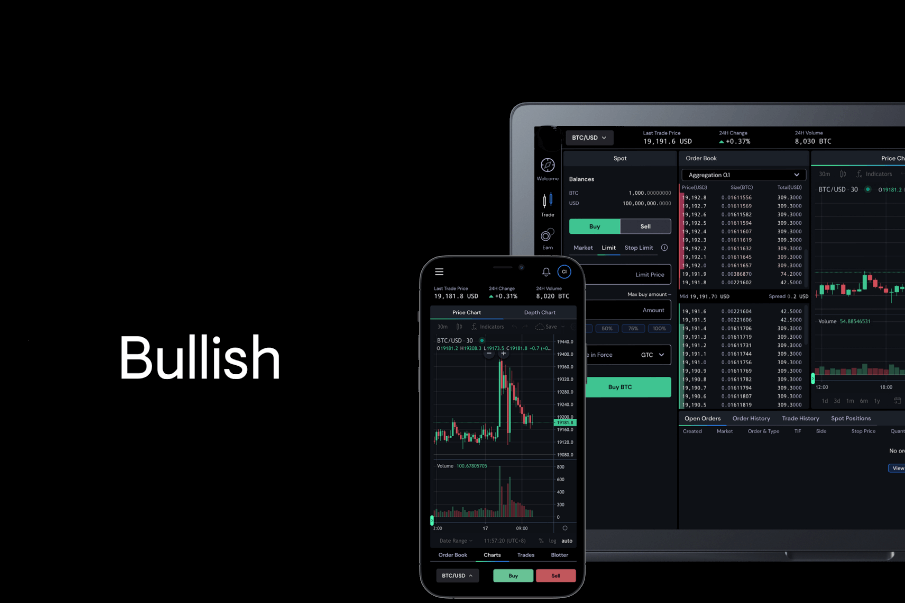# How is Forex deposit interest rate calculated?

2023-06-05Summary:

Foreign currency deposits refer to various bank deposits expressed in convertible foreign currencies, mainly including demand deposit, savings deposits and time deposits in foreign currencies. It is the main manifestation of foreign exchange value. Banks can bring substantial profits through the use of foreign exchange deposits.

oneHow to calculate the foreign exchange deposit interest rate?

(1) The interest rate for foreign currency savings deposits is expressed as an annual interest rate.

(2) The deposit period of foreign currency savings deposits is calculated based on 360 days throughout the year, 30 days per month, and the odd days less than one month are calculated based on the actual deposit days. Calculate the actual shelf life from the beginning to the end.

(3) When calculating interest, the principal is calculated in the main currency of a foreign currency, and no interest is calculated below the main currency.

The interest amount is calculated to the minimum unit of each currency, rounded to the nearest unit.

(4) Foreign currency savings deposits are paid with interest in the original currency. When the secondary currency is insufficient, the remaining balance can be converted into RMB for payment.

(5) Foreign currency fixed-term savings deposits are calculated at the interest rate on the date of deposit, which matures once. If interest rate adjustments occur midway, they will not be calculated in segments. The overdue portion and early withdrawal shall be calculated at the current savings interest rate announced on the withdrawal date.

(6) Current savings deposits are interest settled once a year, and after interest settlement, the interest from the previous year is transferred to the principal. If the account is cleared before the interest settlement date, the interest shall be settled along with the principal.2. What is the meaning of foreign exchange deposit interest rate

Foreign currency deposits refer to various bank deposits expressed in convertible foreign currencies, mainly including demand deposit, savings deposits and time deposits in foreign currencies. It is the main manifestation of foreign exchange value. Banks can bring substantial profits through the use of foreign exchange deposits. The foreign exchange deposit interest rate refers to the interest rate at which a customer deposits various foreign currencies into a bank account under agreed conditions, with the same amount of interest as the loan amount or principal for a certain period of time. The interest rate for US dollar deposits can be divided into demand interest rate and term interest rate, as well as annual/monthly/daily interest rate. The US dollar deposit interest rate will be set by banks based on the market conditions of each country, with certain differences and will not change depending on the type of currency.

3. Formula for calculating insurance interest rate

1、 Basic formula for calculating interest

The basic formula for calculating the interest of savings deposits is: interest=principal × Maturity × Interest rate.

2、 Conversion of interest rates

The conversion relationship between annual interest rate, monthly interest rate, and daily interest rate is: annual interest rate=monthly interest rate × 12 (months)=daily interest rate × 360 days; Monthly interest rate=Annual interest rate ÷ 12 (months)=Daily interest rate × 30 (days); Daily interest rate=annual interest rate ÷ 360 (days)=monthly interest rate ÷ 30 (days). Pay attention to using interest rates that are consistent with the deposit period.

3、 Starting point for interest calculation

1. The starting point for interest calculation on savings deposits is yuan, and no interest will be paid for cents below yuan. 2. The interest amount is calculated to the nearest centimeter, and the actual payment is rounded to the nearest percentile.3. Except for the annual settlement of current savings, which can transfer interest to the principal to generate interest, all other types of savings deposits, regardless of the deposit period, will have their interest paid off along with the principal upon withdrawal, without any compound interest.

4、 Calculation of shelf life

1. The method of calculating the shelf life is to calculate the beginning but not the end.

2. Regardless of the major month, minor month, regular month, or leap month, it is calculated as 30 days per month and 360 days per year.

3. The maturity dates of various deposits are calculated on a year to month basis. If the account opening date is missing from the maturity month, the end of the maturity month is used as the maturity date.

5、 Calculation of interest on foreign currency savings deposits

The interest rate for foreign currency savings deposits shall be implemented in accordance with the interest rate announced by the People's Bank of China, and the original currency savings shall be carried out with interest calculated in the original currency (the secondary currency can be converted into RMB for payment based on the foreign exchange rate on the day). The interest calculation regulations and calculation methods are similar to the RMB savings method.

【 EBC Platform Risk Reminder and Disclaimer 】: There are risks in the market, and investment needs to be cautious. This article does not constitute investment advice.### What does dividend yield mean?

Dividend yield, calculated by dividing annual dividends by the current share price, gauges income from a stock. A high yield suggests stable returns, but consider other factors like cash flow for a complete evaluation.

2023-12-06### What does a long position?

A long position involves holding a bullish stance, anticipating market or asset price increases. Strategies like alignment, divergence, and hedging are employed, with attention to reversal patterns such as head-and-shoulder bottoms.

2023-12-04### What is the status of the volume-price relationship?

The volume-price relationship is a key stock market indicator, revealing the correlation between trading volume and stock prices. Analyzing these changes helps investors understand market activity and potential trend reversals.

2023-12-01